Maths-
General
Easy

Question

# If the tangent at ‘t’ on the curve y =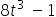, x =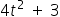meets the curve again at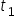and is normal to the curve at that point, then value of t must be -

## ±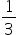±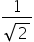±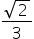None of theseHint:

## The correct answer is: ±### Slope of tangent =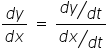Given : y =and x =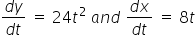=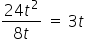Equation tangent at point 't'y -y1 = m(x -x1)Equation of normal#### With Turito Foundation.#### Get an Expert Advice From Turito.